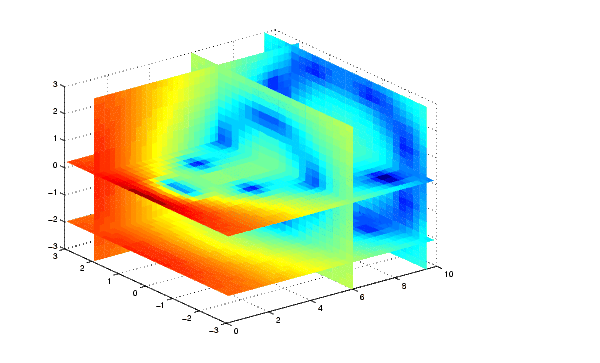MATLAB Function Referenceinterp3

Three-dimensional data interpolation (table lookup)

Syntax

• ```VI = interp3(X,Y,Z,V,XI,YI,ZI)
VI = interp3(V,XI,YI,ZI)
VI = interp3(V,ntimes)
VI = interp3(...,method)
VI = INTERP3(...,'method',extrapval)
```

Description

```VI = interp3(X,Y,Z,V,XI,YI,ZI) ``` interpolates to find `VI`, the values of the underlying three-dimensional function `V` at the points in arrays `XI`,`YI` and `ZI`. `XI`,`YI`, `ZI` must be arrays of the same size, or vectors. Vector arguments that are not the same size, and have mixed orientations (i.e. with both row and column vectors) are passed through `meshgrid` to create the `Y1`, `Y2`, `Y3` arrays. Arrays `X`, `Y`, and `Z` specify the points at which the data `V` is given. Out of range values are returned as `NaN`.

```VI = interp3(V,XI,YI,ZI) ``` assumes `X=1:N`, `Y=1:M`, `Z=1:P` where `[M,N,P]=size(V)`.

```VI = interp3(V,ntimes) ``` expands `V` by interleaving interpolates between every element, working recursively for `ntimes` iterations. The command `interp3(V)` is the same as `interp3(V,1)`.

```VI = interp3(...,method) ``` specifies alternative methods:

 `'linear'` Linear interpolation (default) `'cubic'` Cubic interpolation `'spline'` Cubic spline interpolation `'nearest'` Nearest neighbor interpolation

`VI = INTERP3(...,'method',extrapval)` specifies a method and a value for `VI` outside of the domain created by `X`,`Y` and `Z`. Thus, `VI` equals `extrapval` for any value of `XI`, `YI` or `ZI` that is not spanned by `X`, `Y`, and `Z`, respectively. You must specify a method to use `extrapval`. The default method is `'linear'`.

Discussion

All the interpolation methods require that `X`,`Y` and `Z` be monotonic and have the same format ("plaid") as if they were created using `meshgrid`. `X`, `Y`, and `Z` can be non-uniformly spaced. For faster interpolation when `X`, `Y`, and `Z` are equally spaced and monotonic, use the methods '`*linear`', '`*cubic`', or '`*nearest`'.

Examples

To generate a coarse approximation of `flow` and interpolate over a finer mesh:

• ```[x,y,z,v] = flow(10);
[xi,yi,zi] = meshgrid(.1:.25:10, -3:.25:3, -3:.25:3);
vi = interp3(x,y,z,v,xi,yi,zi); % vi is 25-by-40-by-25
slice(xi,yi,zi,vi,[6 9.5],2,[-2 .2]), shading flat```

See Also

`interp1`, `interp2`, `interpn`, `meshgrid`

© 1994-2005 The MathWorks, Inc.